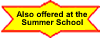# Junior Mathematics and Statistics

This site contains information on Junior Mathematics at the University of Sydney. Each unit of study has its own web page; links to these pages are given in the table below.

Enrolment advice, FAQs and Student Policies can be found on the School of Mathematics and Statistics Student Portal, on the Canvas website, or on the Enrolment Advice page on this server.

Enrolment advice sessions with Director of First Year Studies

Join either or both of the sessions if you are not sure which mathematics or statistics units you should take, for instance for your major or to satisfy the Science core requirements.

## Contact details

General and administrative enquiries about Junior units of study should be directed to the School of Mathematics and Statistics Student Office, who can be contacted at math.schooloffice@sydney.edu.au.

Phone number: 9351 5787.

The Director of First Year Studies in the School of Mathematics and Statistics is Sharon Stephen (Carslaw 525).

Enquiries about details of a particular unit of study should be directed to the lecturer for that unit, who can be contacted by email if necessary. For further information, follow the link below to the web page for the relevant unit.

## Special consideration and special arrangements

Do not apply for special consideration for attendance or quizzes. See the information on the Special Consideration page.

## FAQ

There is a Frequently Asked Questions page. If there is something you want to know, check there first!

## Handbook

The 2021 Junior Mathematics and Statistics Handbook contains a summary of important information.

## Further important information

Students may wish to compete in the Norbert Quirk Mathematical Essay Competition.

## Units of Study 2021

First Semester Second Semester
 MATH1921 Calculus of One Variable (Advanced) MATH1902 Linear Algebra (Advanced) MATH1931 (Special Studies Program) A DATA1901 Foundations of Data Science (Advanced)
 MATH1021 Calculus of One VariableMATH1023 Multivariable Calculus and ModellingMATH1002 Linear Algebra MATH1005 Statistical Thinking about DataDATA1001 Foundations of Data Science
 MATH1021 Calculus of One VariableMATH1023 Multivariable Calculus and ModellingMATH1004 Discrete Mathematics MATH1005 Statistical Thinking about DataDATA1001 Foundations of Data Science MATH1115 Interrogating DataMATH1064 Discrete Mathematics for Computation
 MATH1011 Applications of Calculus MATH1005 Statistical Thinking about DataMATH1013 Mathematical ModellingMATH1014 Introduction to Linear AlgebraMATH1005 Statistical Thinking about Data